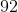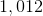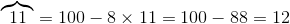# ISEE Lower Level Quantitative : How to subtract

## Example Questions

← Previous 1 3 4 5 6

### Example Question #347 : Operations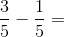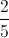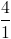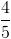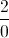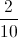Explanation:

To subtract fractions, we first see if they have the same denominators. In this case, the two fractions have the same denominator (5).

Then we subtract the second numerator from the first numerator: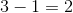.

We keep the original denominator and get. Do not subtract the denominators.

### Example Question #1 : How To Subtract

Sam bought a video game and an MP3 player from the store with money from his birthday.  He spent $264.21, including tax. The following day, he had to return the video game. His new total spent was$197.89 for the MP3 player.  When he returned the video game, how much money did he get back?

$133.68$66.32

$65.32$166.32

$66.32 Explanation: To find the difference, you must subtract. Line up the numbers vertically. Remember to use the rules of borrowing to subtract.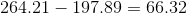Sam got$66.32 back when he returned the video game.

### Example Question #2 : How To Subtract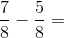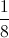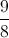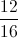Explanation:

Subtract the numerators and keep the denominators the same.

The correct answer is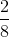or.

### Example Question #350 : Operations

One coin has a thickness ofinches, while another coin has a thickness ofinches. How much thicker is the second coin?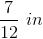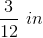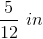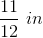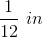Explanation:

To solve this problem, we have to subtract the smaller thickness from the larger thickness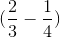.

When subtracting fractions, we must find a common denominator (in this case, 12) and then change the numerators accordingly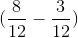.

The answer is therefore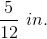### Example Question #351 : Operations

Subtract: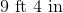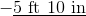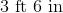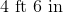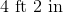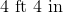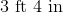Explanation:

One foot is equal to 12 inches, so: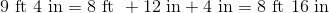In this equivalent problem, subtract feet and inches separately: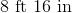### Example Question #2 : How To Subtract

Subtract: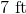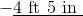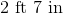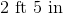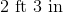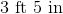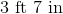Explanation:

One foot is equal to 12 inches, so: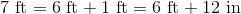In this equivalent problem, subtract feet and inches separately: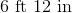### Example Question #353 : Operations

Karen wins a bag of 100 marbles and wants to give some to her sister Kay so that each girl will have the same number of marbles. Karen already has 71 marbles; Kay already has 51.  How many of the 100 marbles will Karen keep?Explanation:

After Janet wins the 100 marbles, the girls together own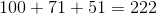marbles. Each should therefore own half this, or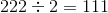marbles. Since Karen owns 71 marbles already, she will keep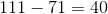marbles.

### Example Question #3 : How To Subtract

Give the difference of 6 and 0.77.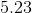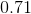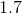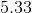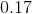Explanation:

Write the two numbers vertically with decimal points aligned, adding a decimal point and two zeroes to the 6, and subtract: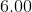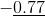### Example Question #4 : How To Subtract

Give the difference of 7.4 and 0.134.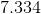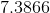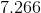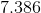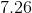Explanation:

Write the two numbers vertically, adding two zeroes to the 7.4, and subtract: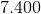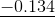### Example Question #5 : How To Subtract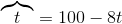Evaluate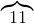.The expression is undefined.